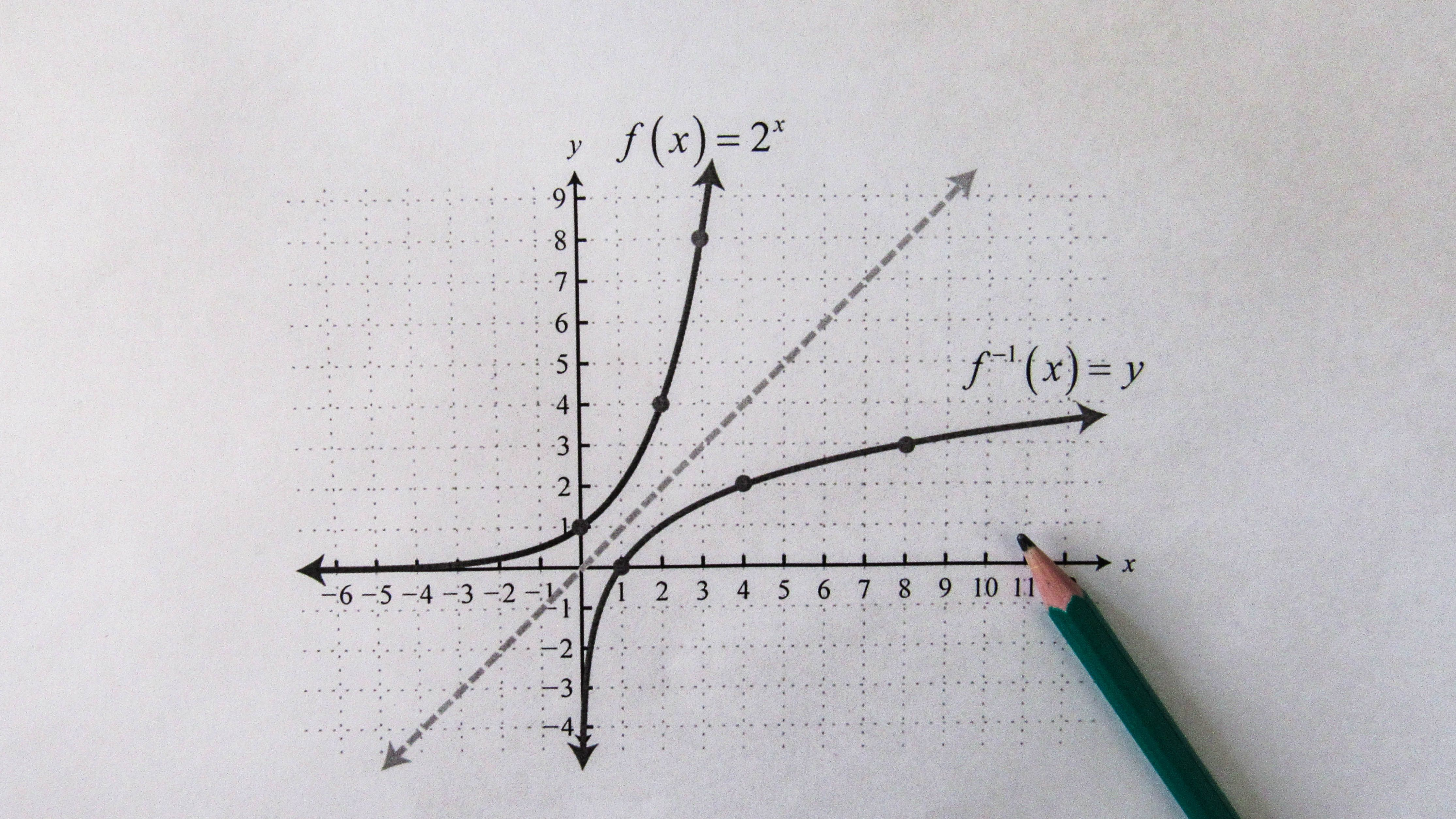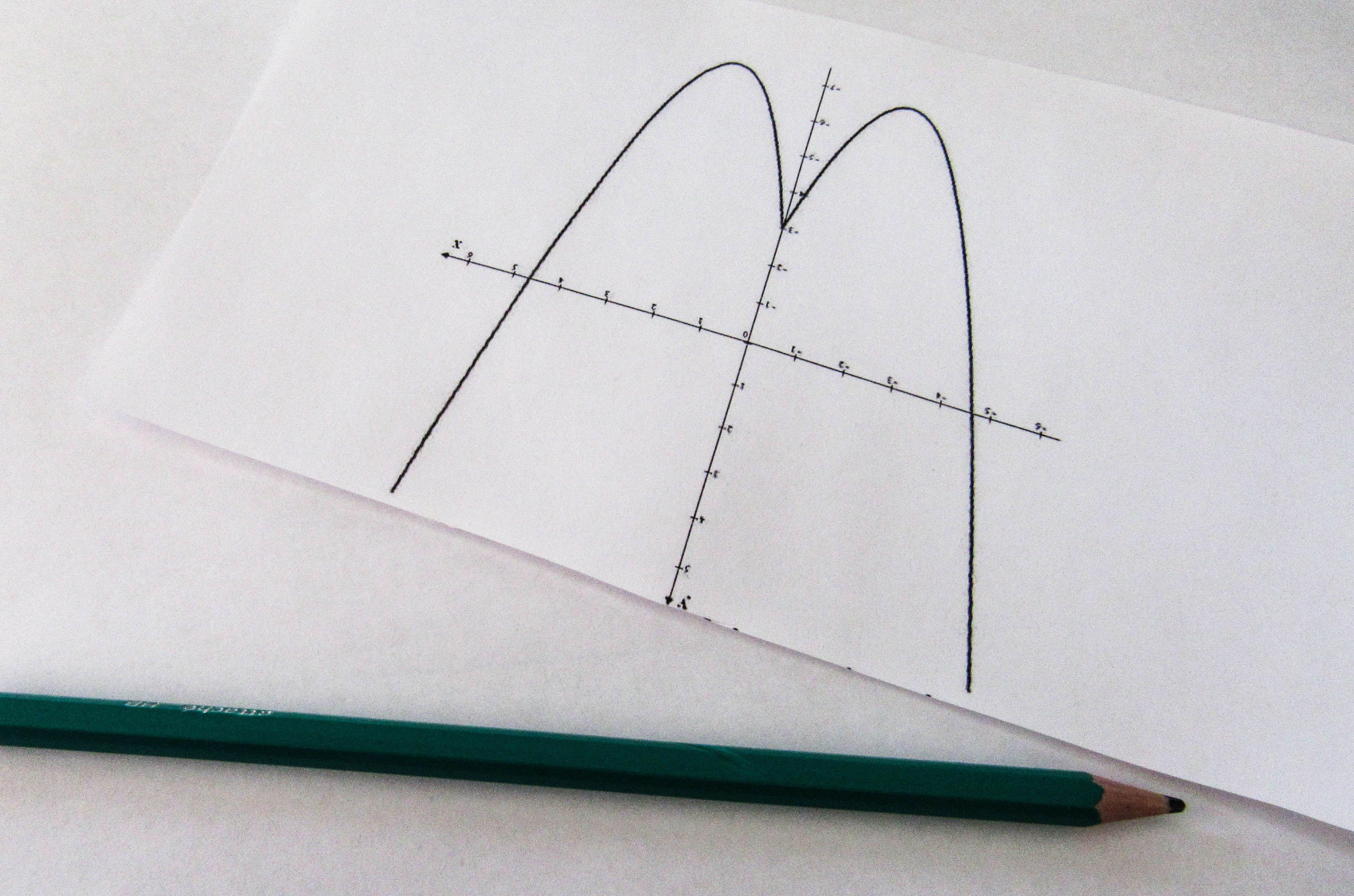# What topics are in finite math?

What Topics Are in Finite Math?

# What Topics Are in Finite Math?Are you interested in studying finite math, but not sure what topics to expect? In this article, we will dive into the major topics covered in finite math. Whether you are a math major or just need to complete a required math course, understanding the concepts in finite math will provide a foundation for a wide range of fields.

## FAQs## Matrix Algebra

Matrix algebra deals with matrices, which are rectangular arrays of numbers, symbols, or expressions. In finite math, matrices are used to represent systems of linear equations, data sets, and transformations in geometry. The basic operations in matrix algebra include addition, subtraction, scalar multiplication, matrix multiplication, and finding the inverse of a matrix.

Example of matrix:

| 3 4 5 |

| 1 2 3 |

| 0 1 3 |## Linear Programming

Linear programming is a method of optimization where a mathematical model is used to determine the best solution to a problem. In finite math, linear programming is used to solve problems related to resource allocation, production planning, and transportation. Linear programming involves creating a set of linear inequalities, or constraints, that define the feasible region of solutions, and finding the optimal solution that maximizes or minimizes a given objective function.## Probability

Probability is the branch of mathematics that deals with the study of chance and randomness. In finite math, probability is used to model and analyze experiments that involve uncertainty, such as flipping a coin, rolling a dice, or drawing a card from a deck. The basic concepts in probability include sample space, events, probability measures, conditional probability, and independent events.

## Counting Methods

Counting methods are used to count the number of ways to select or arrange objects. In finite math, counting methods are used to solve problems related to probability, coding theory, and finance. The basic counting methods include permutations, combinations, and the multiplication principle.

## Markov chains

Markov chains are mathematical models that describe a sequence of events where the probability of each event depends only on the previous event. In finite math, Markov chains are used to model systems that exhibit probabilistic behavior, such as stock prices, weather patterns, and traffic flow. The basic concepts in Markov chains include state transitions, transition matrices, and steady-state analysis.

## Game Theory

Game theory is the study of strategic decision-making in situations where the outcome of a decision depends on the decisions of other players. In finite math, game theory is used to analyze conflicts and cooperation in economics, politics, and social interactions. The basic concepts in game theory include game forms, strategies, and equilibria.